# Introduction To Depth-First Search

suggest change

Depth-first search is an algorithm for traversing or searching tree or graph data structures. One starts at the root and explores as far as possible along each branch before backtracking. A version of depth-first search was investigated in the 19th century French mathematician Charles Pierre Trémaux as a strategy for solving mazes.

Depth-first search is a systematic way to find all the vertices reachable from a source vertex. Like breadth-first search, DFS traverse a connected component of a given graph and defines a spanning tree. The basic idea of depth-first search is methodically exploring every edge. We start over from a different vertices as necessary. As soon as we discover a vertex, DFS starts exploring from it (unlike BFS, which puts a vertex on a queue so that it explores from it later).

Let’s look at an example. We’ll traverse this graph: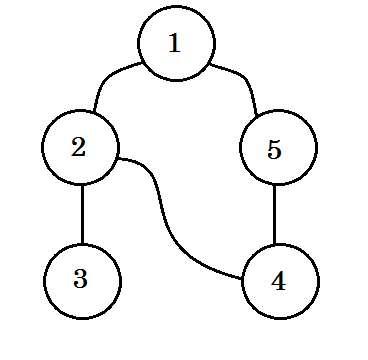We’ll traverse the graph following these rules:

• We’ll start from the source.
• No node will be visited twice.
• The nodes we didn’t visit yet, will be colored white.
• The node we visited, but didn’t visit all of its child nodes, will be colored grey.
• Completely traversed nodes will be colored black.

Let’s look at it step by step: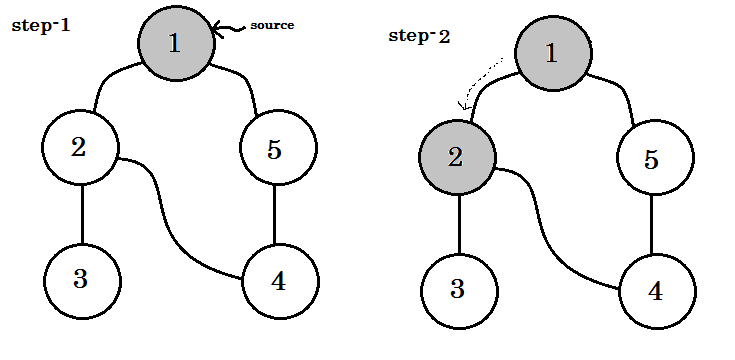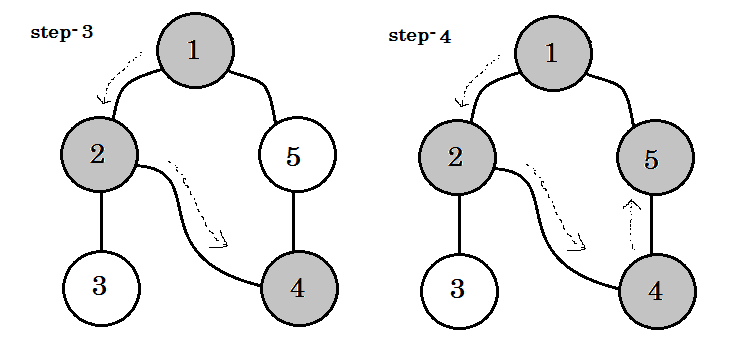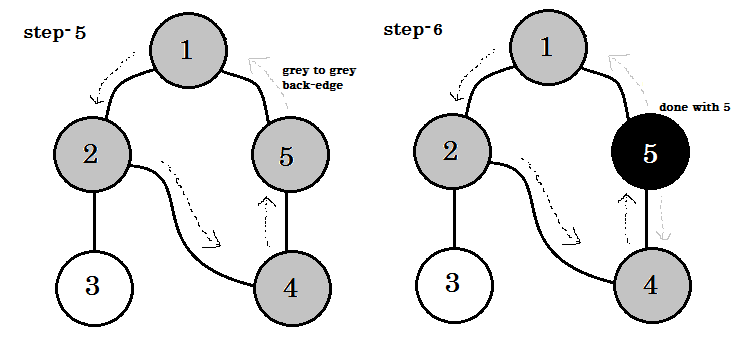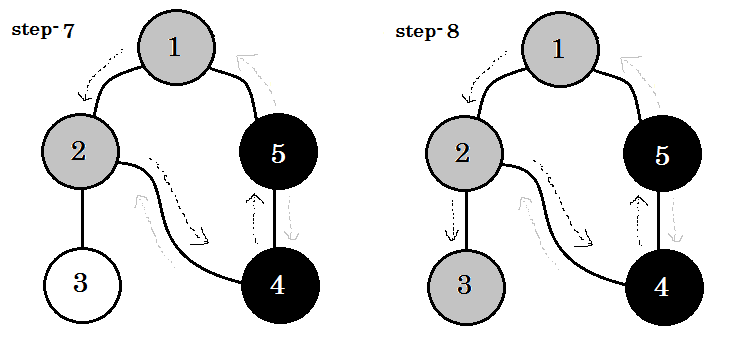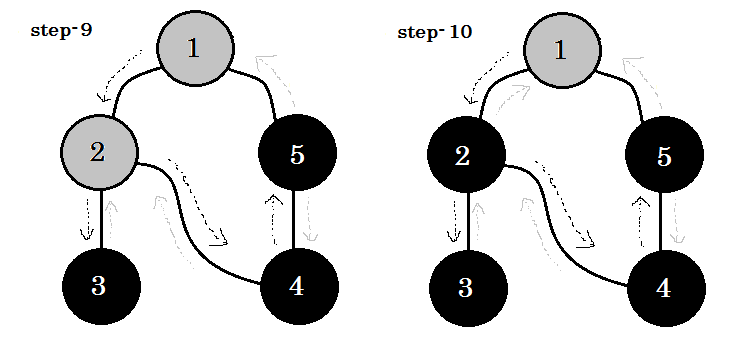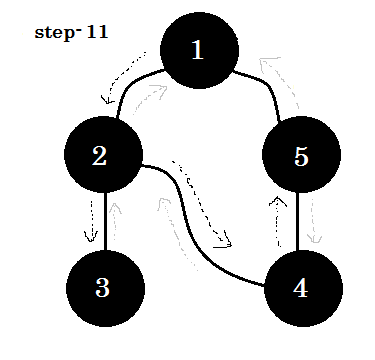We can see one important keyword. That is backedge. You can see. 5-1 is called backedge. This is because, we’re not yet done with node-1, so going from another node to node-1 means there’s a cycle in the graph. In DFS, if we can go from one gray node to another, we can be certain that the graph has a cycle. This is one of the ways of detecting cycle in a graph. Depending on source node and the order of the nodes we visit, we can find out any edge in a cycle as backedge. For example: if we went to 5 from 1 first, we’d have found out 2-1 as backedge.

The edge that we take to go from gray node to white node are called tree edge. If we only keep the tree edge‘s and remove others, we’ll get DFS tree.

In undirected graph, if we can visit a already visited node, that must be a backedge. But for directed graphs, we must check the colors. If and only if we can go from one gray node to another gray node, that is called a backedge.

In DFS, we can also keep timestamps for each node, which can be used in many ways (e.g.: Topological Sort).

1. When a node v is changed from white to gray the time is recorded in d[v].
2. When a node v is changed from gray to black the time is recorded in f[v].

Here d[] means discovery time and f[] means finishing time. Our pesudo-code will look like:

``````Procedure DFS(G):
for each node u in V[G]
color[u] := white
parent[u] := NULL
end for
time := 0
for each node u in V[G]
if color[u] == white
DFS-Visit(u)
end if
end for

Procedure DFS-Visit(u):
color[u] := gray
time := time + 1
d[u] := time
for each node v adjacent to u
if color[v] == white
parent[v] := u
DFS-Visit(v)
end if
end for
color[u] := black
time := time + 1
f[u] := time``````

Complexity:

Each nodes and edges are visited once. So the complexity of DFS is O(V+E), where V denotes the number of nodes and E denotes the number of edges.

Applications of Depth First Search:

• Finding all pair shortest path in an undirected graph.
• Detecting cycle in a graph.
• Path finding.
• Topological Sort.
• Testing if a graph is bipartite.
• Finding Strongly Connected Component.
• Solving puzzles with one solution.